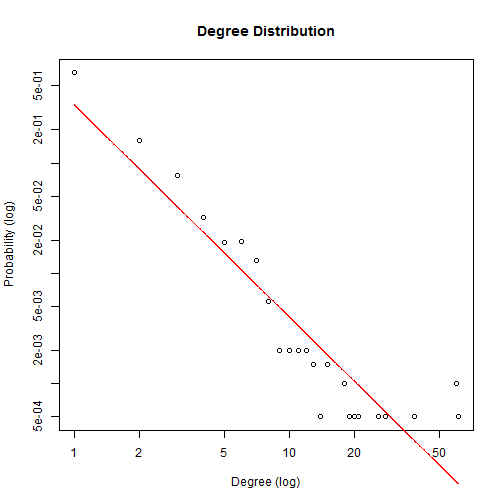# Simulate networks and fit the power law distribution

``````# install.packages('igraph')

library(igraph)
size = 50

# tree graph with two children for each node
g = graph.tree(size, children = 2)
plot(g)
``````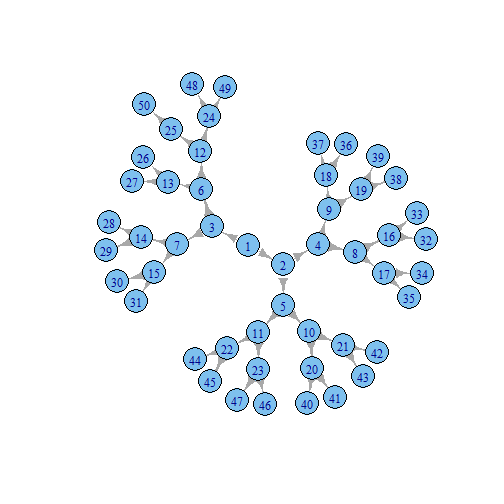``````# star network
g = graph.star(size)
plot(g)
``````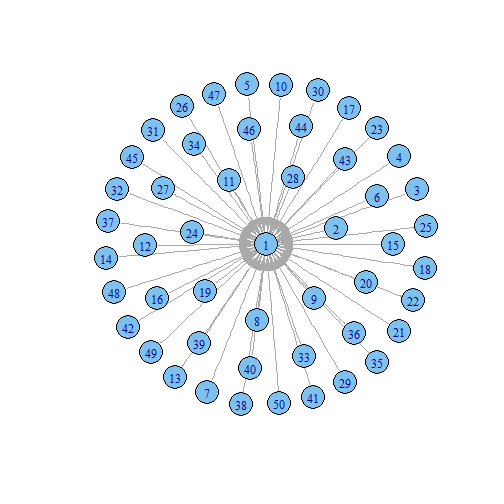``````# full network
g = graph.full(size)
plot(g)
``````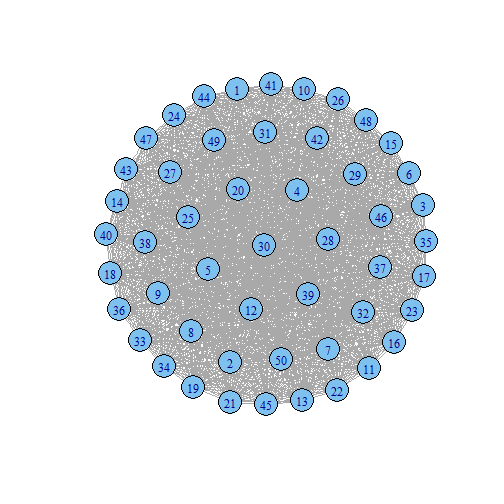``````# ring network
g = graph.ring(size)
plot(g)
``````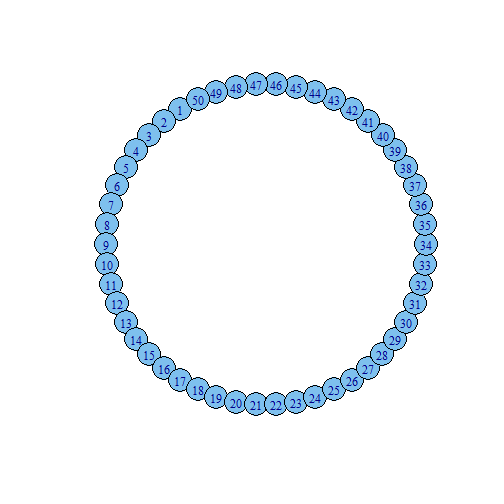``````# connect the node with the vertices not farther than a given limit
g = connect.neighborhood(graph.ring(size), 4)
plot(g)
``````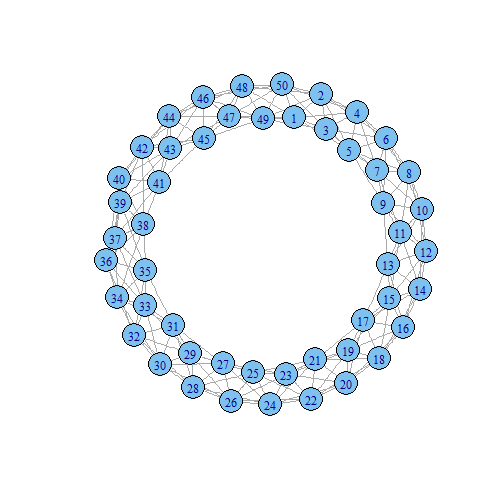``````
# random network
g = erdos.renyi.game(size, 0.1)
plot(g)
``````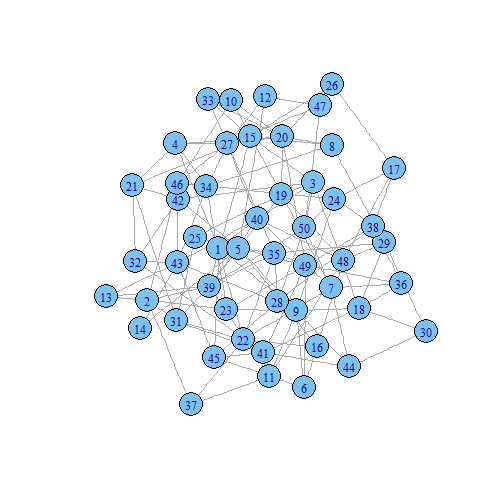``````
# small-world network
g = rewire.edges(erdos.renyi.game(size, 0.1), prob = 0.8)
plot(g)
``````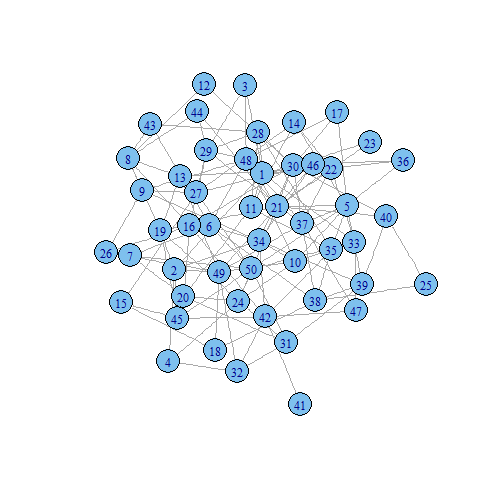``````# scale-free network
g = barabasi.game(size)
plot(g)
``````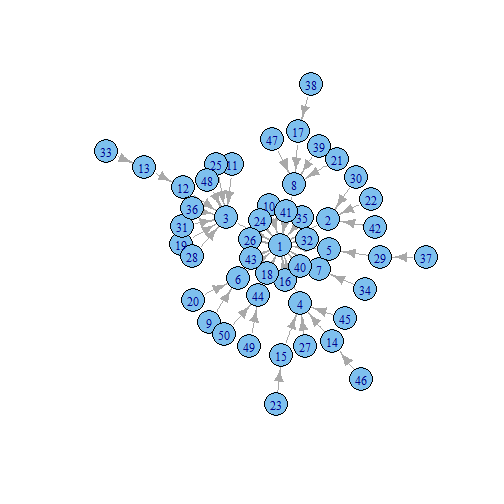``````
# degree and degree distribution
d = degree(g, mode = "all")
hist(d)
``````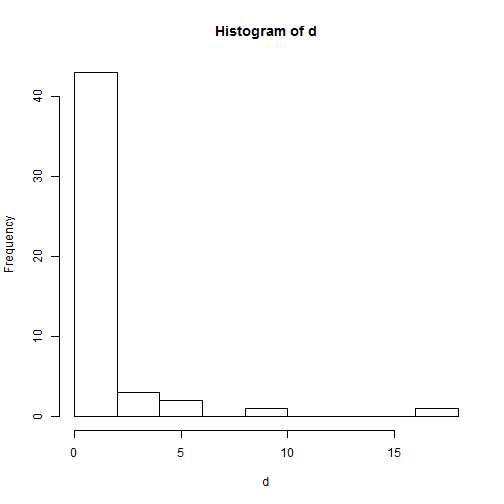``````dd = degree.distribution(g, mode = "all", cumulative = FALSE)

# Plot degree distribution

# write a function to plot the degree distribution
plot_degree_distribution = function(graph) {
# calculate degree
d = degree(graph, mode = "all")
dd = degree.distribution(graph, mode = "all", cumulative = FALSE)
degree = 1:max(d)
probability = dd[-1]
# delete blank values
nonzero.position = which(probability != 0)
probability = probability[nonzero.position]
degree = degree[nonzero.position]
# plot
plot(probability ~ degree, log = "xy", xlab = "Degree (log)", ylab = "Probability (log)",
col = 1, main = "Degree Distribution")
}

plot_degree_distribution(g)
``````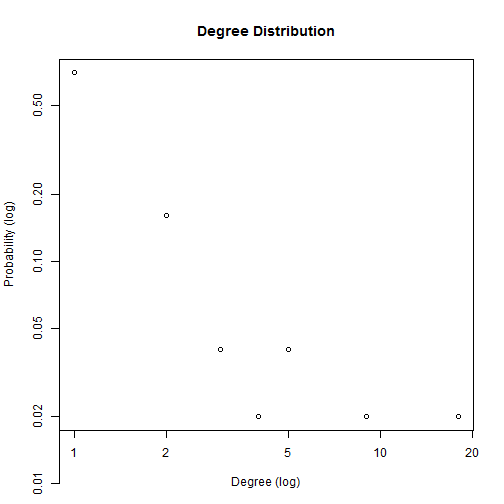``````
g.big.ba = barabasi.game(2000)
g.big.er = erdos.renyi.game(2000, 0.1)

plot_degree_distribution(g.big.ba)
``````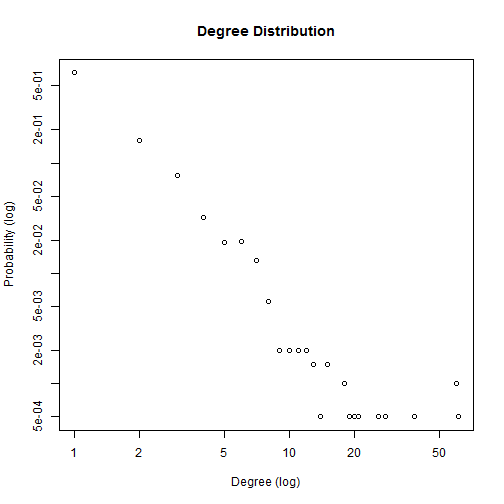``````plot_degree_distribution(g.big.er)
``````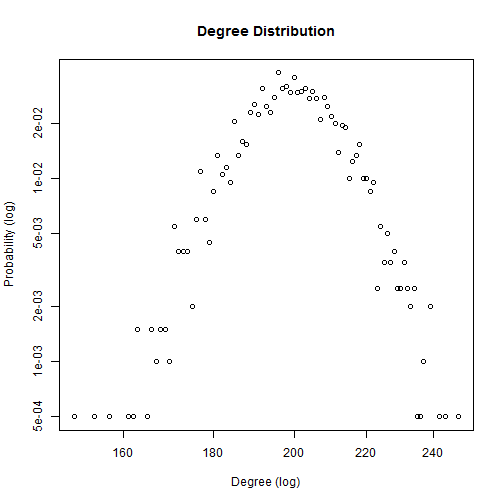``````

# plot and fit the power law distribution
fit_power_law = function(graph) {
# calculate degree
d = degree(graph, mode = "all")
dd = degree.distribution(graph, mode = "all", cumulative = FALSE)
degree = 1:max(d)
probability = dd[-1]
# delete blank values
nonzero.position = which(probability != 0)
probability = probability[nonzero.position]
degree = degree[nonzero.position]
reg = lm(log(probability) ~ log(degree))
cozf = coef(reg)
power.law.fit = function(x) exp(cozf[] + cozf[] * log(x))
alpha = -cozf[]
R.square = summary(reg)\$r.squared
print(paste("Alpha =", round(alpha, 3)))
print(paste("R square =", round(R.square, 3)))
# plot
plot(probability ~ degree, log = "xy", xlab = "Degree (log)", ylab = "Probability (log)",
col = 1, main = "Degree Distribution")
curve(power.law.fit, col = "red", add = T, n = length(d))
}

fit_power_law(g.big.ba)
``````
``````##  "Alpha = 1.925"
##  "R square = 0.858"
``````